# What Makes Ellipses… Ellipses?

Last time we used wild properties of ellipses to build some really easy—and some really devilish—golf courses. Specifically, I claimed that every ellipse has two magical points F1 and F2 (called foci) such that a ray from F1 always bounces off the ellipse and lands precisely at F2, and furthermore, this path always has the same length. Why does this happen? And how do we find these foci?

Let’s focus(!) on the last question first. Recall that an ellipse is a stretched circle. In other words, an ellipse is what forms when you slice a tall, circular tube (cylinder) along a slant: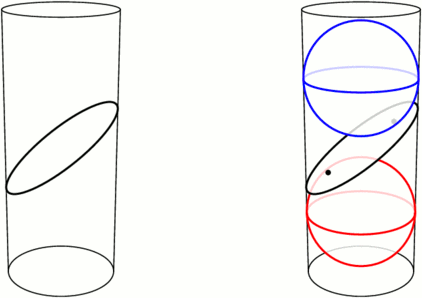Left: An ellipse is formed by slicing a cylinder. Right: Fitting spheres above and below the cut locates the ellipse’s foci.

Take a sphere that snugly fits inside the tube, and drop it down until it touches the ellipse-slice at a single point F1. Do the same with a sphere underneath, touching the slice at F2. These points turn out to be the foci of the ellipse. Let’s see why.

We can use this tubular setup to answer one mystery from earlier: for any point X on the ellipse, the sum of distances of XF1 and XF2 is always the same! The proof lies in the following animation. Segments XF1 and XA have the same length because they’re both tangent to the upper sphere from X, and similarly, XF2=XB. So the sum XF1+XF2 is just the length of segment AB, the height between the spheres’ equators.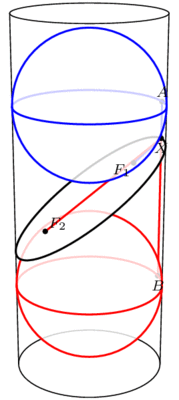The sum F1X+XF2 equals the length of AB and therefore does not change when X moves.

This has two neat consequences. First, it provides an elementary method for drawing ellipses (in real life!): all you need are two push pins and a loop of string, as illustrated below. The string ensures that the sum XF1+XF2 stays fixed while you trace the pen around, as long as you’re careful to keep the string taut throughout.How to draw an ellipse with push-pins and string

Second, what happens if we slice a cone instead of a cylinder? Perhaps surprisingly, we still get an ellipse! Indeed, as above, we can create a sphere on either side of the slice that snugly fits against the slice and the walls of the cone (the so-called Dandelin spheres), and exactly the same proof shows that XF1+XF2 stays constant as X moves around the edge.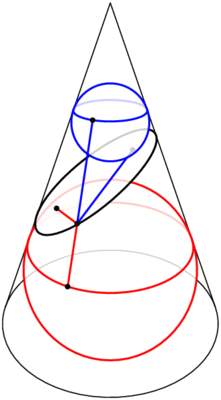Slicing a cone also produces an ellipse, by the same argument.

But wait, there’s still an unanswered question! We’ve seen that the path F1X+XF2 has a fixed length, but why does light bounce off an ellipse along such a path? This is what we really cared about for mini-golf! Come back next time for the answer, and in the meantime, have a great 2 weeks.

# More Putting Predicaments

Last time we discussed some rather challenging holes of (mathematical) mini-golf, uncovering Tokarski’s construction of some un-hole-in-one-able holes. By contrast, here is the all-time easiest hole of mini-golf, namely, a guaranteed hole-in-one: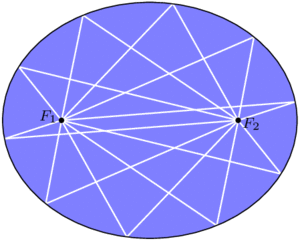In an ellipse, any golf shot from focus $$F_1$$ will bounce directly to the other focus, $$F_2$$.

By some miracle of geometry, if we take an ellipse (i.e., a stretched or squashed circle) and place the ball and cup at two special points $$F_1$$ and $$F_2$$ called the foci of the ellipse, then any golf shot leaving $$F_1$$ will bounce off a wall and proceed directly to $$F_2$$. You can’t miss!

Curiously, this easiest golf hole can be used to construct some even more challenging ones. For starters, consider a mushroom-shaped room as illustrated below (left), where the “mushroom head” is half of an ellipse with foci $$F_1$$ and $$F_2$$. Then the same reflection principle mentioned above tells us that any shot entering the mushroom head via segment $$F_1F_2$$ will be reflected straight back through $$F_1F_2$$ and sent back down the stem. This means that no shot from P can ever reach the triangular “fang” containing Q, and in particular, no hole-in-one from P to Q is possible. In the mirrored-room setting (described last time), if a light source is placed at P, then the whole triangular fang remains unilluminated! This is stronger than Tokarski’s room, where only a single point remained unlit. Similar constructions are possible even with rooms that have no corners, such as the “curvy” mushroom on the right.Left: Any path entering the mushroom head from segment $$F_1F_2$$ will be reflected back down through this segment, so the triangle containing Q is not reachable from P. Right: The same phenomenon is possible even when the room has no corners.

With this idea, we can construct a mirrored room that has dark patches no matter where a light bulb is placed. In the image below, if a light source is placed anywhere in the top half of the room, the lower triangular “fang” will be completely dark. Similarly, the upper fang is not illuminated from anywhere in the bottom half of the room. This room (or one quite like it) was originally designed by Roger Penrose in 1958.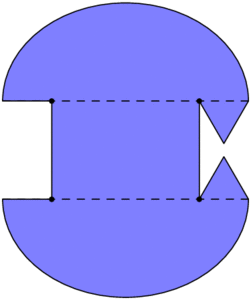This room will have dark regions no matter where the light source is placed.

Back in the mini-golf setting, we can do something even more devious: by chaining multiple Penrose rooms together, we can construct golf holes that require as many shots as we wish! The golf hole below cannot be completed with fewer than 7 shots. Indeed, no single shot can cross two dashed segments with different numbers, so 7 shots are required.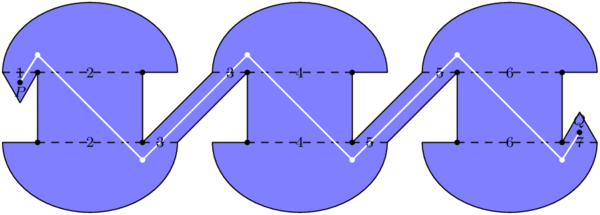At least 7 shots are required to get from P to Q.

### What about polygons?

The golf holes last time were all polygons, whereas we have allowed curved boundaries here. Can we construct a polygonal golf hole, using only flat walls, that still requires at least 7 shots? Or even 3? Unfortunately, the answer this time is “we don’t know, but we think not.” In particular, it has been conjectured that, no matter where the ball and cup are placed in a polygonal golf hole, a single shot can place the ball as close to the hole as desired, so a short second putt can finish the job.

### Notes

1. In fact, even more is true: all of these paths from $$F_1$$ to $$F_2$$ have the same length! We will not prove these facts today. []
2. Assuming you hit hard enough, of course. []
3. For the analysts out there, the room’s boundary can be made smooth. []
4. This too can be accomplished with smooth boundary. []
5. More strongly, O’Rourke and Petrovici conjecture that with a single light source in a polygonal room, the set of unilluminated points has measure 0. Reference: Joseph O’Rourke and Octavia Petrovici. Narrowing Light Rays with Mirrors. Proceedings of the 13th Canadian Conference on Computational Geometry, 137–140, 2001. []

# How Unilluminating!

You are standing in a large, oddly-shaped room, all of whose walls are lined with mirrors. Everything is dark except for a single light bulb somewhere else in the room. The light from this bulb spreads out in all directions and bounces off the mirrors, possibly many times over. Is it possible that, despite all the mirrors, you could still be standing in the dark, unable to see light from this bulb from any direction?

Said differently, consider the room as a large hole of mathematical mini-golf, where the locations of the ball (just one point) and cup (also just a point) are predetermined. Your goal is to pick a direction to hit the ball, hoping that it will reflect off the walls and eventually find its way to the cup. Is a hole-in-one always possible?When a light ray hits a wall, it bounces off in such a way that the angle of incidence (top left) equals the angle of reflection (top right). In other words, the dashed trajectory is reflected through the line of the mirror.

We assume that when a ray of light (i.e., the golf ball) hits a mirror, it reflects perfectly, as illustrated above. Let’s also assume that it never loses energy, so it will continue as far as necessary to reach its destination. There’s one corner case to consider, namely, what happens if the ray hits a corner of the room? Well, it’s (usually) ambiguous as to where it should reflect next, so let’s say that the ray just dies if it hits a corner. In the mini-golf formulation, our hole-in-one must avoid the hole’s corners.

With these rules in place, it turns out that it is possible to be left in the dark, or to make a hole-in-one impossible! One of the first examples, provided by Tokarski in 1995, is illustrated below; let’s call this room R.No light ray from P can exactly reach Q.

Let’s see why this room R works, i.e., why point Q is not illuminated by a light source at P.

Notice that R is built from identical square cells. As our ray of light continues through the room, we can keep track of where it is in its current cell—but not which cell it is in—by imagining that the ray is just bouncing around in a single square, as illustrated below. Call this single square S. We are essentially folding up the light ray’s trajectory into S.An example light ray reflecting off the walls in Tokarski’s room, and the corresponding “folded” trajectory in a single cell (top middle). This ray gets very close to Q, but it will never reach it exactly. The grid is colored in a repeating pattern where each cell has 1 light yellow corner and three dark purple corners.

Color the grid points with light yellow and dark purple as illustrated above; in particular, P and Q are both yellow. Tokarski’s room has the interesting property that every dark purple point is in fact a corner of the room, so if the ray hits there, it dies. This means that the corresponding ray in square S would die when hitting one of the purple corners. So, in order for the light to reach Q in Tokarski’s room, the corresponding path in S must start at the yellow corner, end back at this same corner, and completely avoid the purple corners of the square. I claim this is impossible. Why?

Just as we folded room R into a single square, let’s consider unfolding square S to cover the whole plane. Because we’ve assumed perfect reflections, our trajectory in cell S unfolds to a straight line!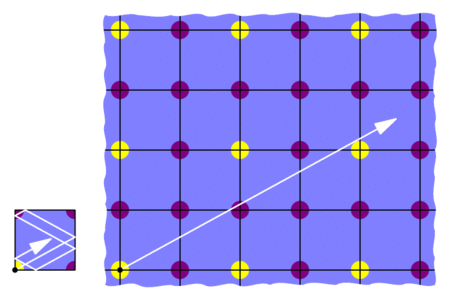“Unfolding” the the light’s trajectory in the square produces a straight path in an infinite grid of squares.

This means that we would need to find a straight trajectory from the initial yellow point to some other yellow point that does not hit any purple points. But this is impossible: all the yellows are blocked by purples! So the light can’t get from P to Q in Tokarski’s room, as claimed.

This room R is not the only example of an unilluminable room; with similar methods, many such rooms may be constructed. For example, in an effort to find an unilluminable room with the smallest number of edges, Tokarski provided the 26-sided polygon below on the left, which was then improved by Castro to a 24-sided polygon on the right. Both are built from 45-45-90 triangles instead of square cells.Tokarski’s 26-sided unilluminable polygon (left) and a 24-sided modification by Castro (right).

Tokarski also provides the gem below (with no right angles!), and his paper gives recipes for a wide variety of other shapes.Another of Tokarski’s unilluminable rooms, this time with no right angles. The basic unit here is a triangle with angles 9, 72, and 99 degrees.

Here’s a challenge: so far, we’ve seen that it is possible to design a mathematical mini-golf hole that requires at least 2 shots. Can we make an even harder hole? Can we design one that needs at least, say, 10 shots? We’ll discuss this next time. See you then!

### Notes

1. George W. Tokarsky. Polygonal Rooms Not Illuminable from Every Point. American Mathathematical Monthly, 102:867–879, 1995. []
2. Here’s a proof: given any yellow-yellow segment, its midpoint lies on the grid. If this midpoint is purple, then we’re done. Otherwise, take half of the original segment and repeat this argument. []
3. D. Castro. Corrections. Quantum 7:42, 1997. []
4. It is not known whether 24 is minimal. []

# Perplexing Paperclips

[I have spent the last few weeks worth of expository writing preparing the content (linked to) in this post, hence the longer-than-usual wait. Enjoy!]

Here’s a rather complicated collection of 54 loops:No two loops link through each other, and yet, these loops really don’t want to come apart. How hard is it to separate them? Well, suppose they were made of thin, bendable string, and we could move and (un)tangle them as much as we wanted as long as we kept the strings intact. Could we separate them? Absolutely not!

What if we allowed ourselves to cross strands through each other—real string can’t do this, of course. Then we could certainly get the loops apart, but how many such crossings would we need to use? As it turns out, we would need at least 108 strand crossings! This is a good first approximation of how much these loops don’t want to separate.

If the strands had open ends, then there would be no such knot-theoretic obstructions to building this shape. So we could, for example, make it with paperclips! (This is not easy…)

I call this Pair o’ Boxes, and it is one of seven new sculptures that I have recently uploaded to my Mathematical Sculpture page. More of these sculptures are shown below, and each has a detailed mathematical description (and larger pictures) at my sculpture page. Go check them out! The Pair o’ Boxes page in particular explains much more about the knot theory of the above structure, including a proof that 108 crossings are necessary to fully separate the strands.Sorry for the shameless advertising; we now return to our regularly-scheduled posting.

# Three-Cornered Deltoids

[This is the third and final post in this series on triangle geometry. See the previous posts on Morley’s theorem and the 9-point circle.]

For our final exploration in this series, let’s again begin with our triangle ABC and a point P on the circumcircle of the triangle, i.e., the circle through the three vertices. If we drop P directly onto the three lines of the triangle at right angles, then by coincidence, these three points lie on a single line, called the Simson line of point P.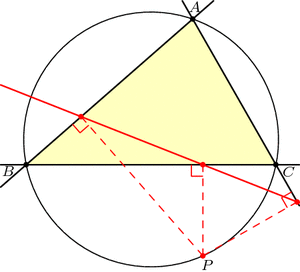Just for fun, let’s draw point Q diametrically opposite from P on the circumcircle, and let’s also look at Q‘s Simson line. How do these two Simson lines interact? Somewhat surprisingly, these lines intersect at right angles. The phenomenal part is that this point of intersection lies on the 9-point circle!Furthermore, as P and Q move around the circumcircle, the intersection of their Simson lines moves around the 9-point circle at twice the speed and in the opposite direction: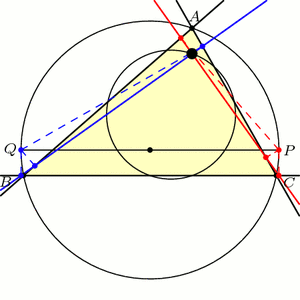This story gets even more unbelievable when we look at how the Simson lines move in this animation. As it turns out, the Simson lines trace a curve in the shape of a deltoid, which is like an equilateral triangle with curved sides. The deltoid traced here is called Steiner’s deltoid.And finally, here’s an incredible fact that ties everything together: If we draw the equilateral triangle around this deltoid, then the edges are parallel to the edges of the equilateral Morley triangle(s).Holy Morley!

### Notes

1. Note that we may have to extend the lines beyond the triangle. []
2. Specifically, a deltoid is the shape that results when you roll a circle inside a circle three-times larger and trace the path of a single point. See Wikipedia:Deltoid_curve for more information. []

# Several Sneaky Circles

In last week’s exploration we uncovered many equilateral triangles hiding in a general triangle ABC. This week we’ll find circles.

Draw any triangle ABC. The line AHA perpendicular to BC is called the altitude though A, with HA at the foot of the altitude. (See the image below.) It can be shown that the three altitudes AHA, BHB, and CHC meet at a single point, H, called the orthocenter of triangle ABC.

Now, let’s put dots at a few important points on our triangle: First, dot the three altitude feet, HA, HB, and HC. Next, dot the midpoints of the sides of the triangle, which we’ll call MA, MB, and MC. Finally, dot the Euler points EA, EB, and EC, which are halfway between H (the orthocenter) and the vertices of our triangle. Today’s first miracle is that all nine of these dots lie on a single circle, aptly named the 9-point circle: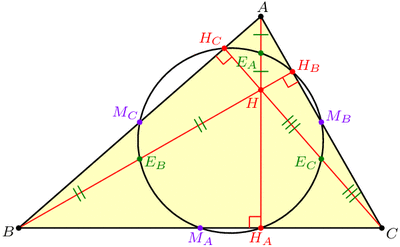The 9-point circle of a triangle is the circle that miraculously passes through the triangle's altitude feet, edge midpoints, and Euler points.

Recall that it only takes three points to determine a circle, so nine on a single circle is very far from coincidence!

Another way to coax a circle out of triangle ABC is to “inscribe” one in the triangle by drawing a circle tangent to the three sides. This circle is called the incircle. Our second miracle is that the incircle and the 9-point circle, which were constructed in very different ways, nevertheless play nicely together: they are tangent! The point where they touch is known as the Feuerbach point of triangle ABC, named after Karl Feuerbach who discovered and proved this difficult fact in 1822.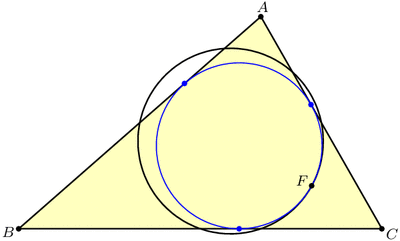The 9-point circle and the incircle are miraculously tangent at the Feuerbach point.

But there are three other circles that, like the incircle, are tangent to all three lines of the triangle. These “exscribed” circles are called the excircles of triangle ABC, and as luck (or extraversions) would have it, these are also tangent to the 9-point circle: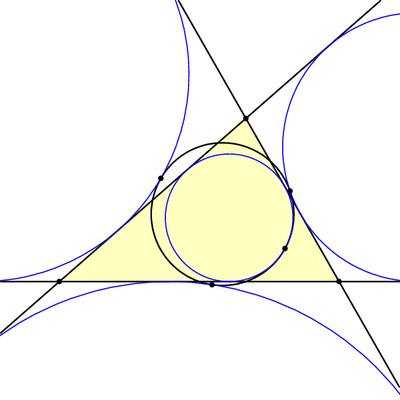The 9-point circle is also tangent to the excircles.

But wait, there’s more! It turns out that triangles ABC and HBC have the same 9-point circle, and so if we look at the incircle and excircles of triangle HBC, these will also be tangent to our 9-point circle. The same is true for triangles HCA and HAB, so we have found 16 circles all tangent to the nine-point circle!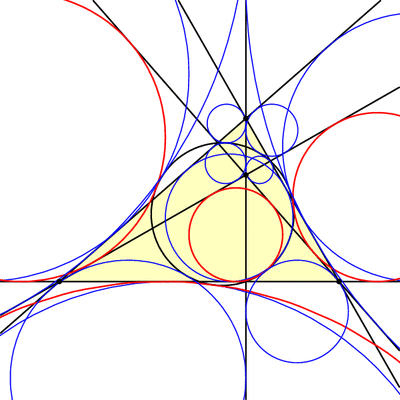The 9-point circle is tangent to the incircle and excircles of triangles ABC, HBC, HCA, and HAB. These four circles for triangle HBC are shown in thick red.

### Notes

1. Recall that we used extraversions last week to go from one Morley triangle to another. Here, extraversions interchange the incircle and excircles. []

# Many Morley Triangles

Given my blog title, how have I gone this long without discussing triangle geometry? I will rectify this gross negligence in the next few weeks. Let’s begin with a seemingly impossible fact due to Frank Morley.

Start with a triangle ABC, with any shape you wish. Now cut its angles into three equal parts, and extend these angle trisectors until they meet in pairs as illustrated below, forming triangle PQR. Morley’s amazing theorem says that this Morley triangle, PQR, will always be equilateral!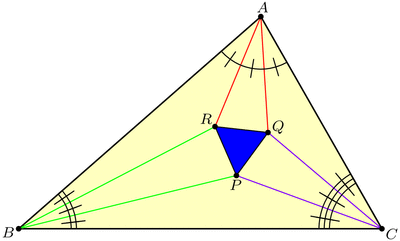Why would this be true? Well, Morley’s theorem tells us that this diagram has three nice 60-degree angles in the middle, but we may suspect that, in fact, all of the angles are nice! This key insight lets us piece together the following argument, where we build up the diagram backwards from its constituent pieces. Draw seven separate “puzzle piece” triangles with angles and side-lengths as shown below, where the original triangle ABC has angles $$3\alpha,3\beta,3\gamma$$ respectively. (Be sure to check that puzzle pieces with these specifications actually exist! Hint: use the Law of Sines.)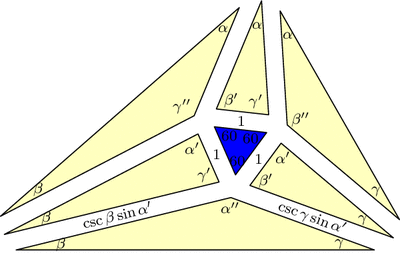Triangle ABC is built out of seven "puzzle pieces" with angles and side-lengths as specified here. In this diagram, $$\alpha^\prime=\alpha+60$$ and $$\alpha^{\prime\prime}=\alpha+120$$, and similarly for $$\beta$$ and $$\gamma$$.

Now, fit the pieces together: all matching edge-lengths are equal (by design), and the angles around vertex P add up to $$60+(\gamma+60)+(\alpha+120)+(\beta+60)=360$$ and similarly for Q and R, so the puzzle fits together into a triangle similar to our original triangle ABC. But now these pieces must make up the Morley configuration, and since we started with an equilateral in the middle, we’re done with the proof!

But there’s more to the Morley story. Let’s push A and C to make them swap positions, dragging the trisectors and Morley triangle along for the ride (a process known as extraversion). We end up using some external angle trisectors instead of only internal ones, but the Morley triangle remains equilateral throughout. This gives us a new equilateral Morley triangle for our original ABC!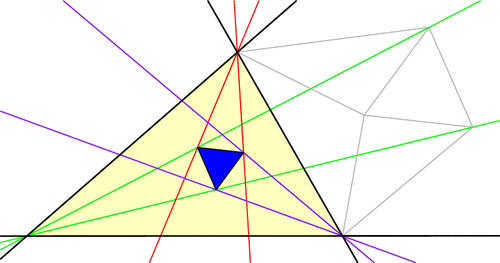In fact, each vertex of ABC has six angle trisectors (three pairs of two), and if you continue applying extraversions you’ll soon uncover that our original triangle ABC has 18 equilateral Morley triangles arranged in a stunning configuration! (Click for larger image.)Here’s a challenge: the diagram above has 27 equilateral triangles, but only 18 of them are Morley triangles (i.e., are made from a pair of trisectors from each vertex). Which ones are they?

### Notes

1. This is a slight modification of a proof by Conway and Doyle. []

# Seeing Stars

Let us now take a closer look at the hex-fractal we sliced last week. Chopping a level 0, 1, 2, and 3 Menger sponge through our slanted plane gives the following:Studying the slicings of various Menger sponge levels gives an algorithm for describing the hex-fractal.

This suggests an iterative recipe to generate the hex-fractal. Any time we see a hexagon, chop it into six smaller hexagons and six triangles as illustrated below. Similarly, any time we see a triangle, chop it into a hexagon and three triangles like this:A recipe for constructing the hex-fractal: replace each hexagon with 6 hexagons and 6 triangles, and replace each triangle with 1 hexagon and 3 triangles.

In the limit, each triangle and hexagon in the above image becomes a hex-fractal or a tri-fractal, respectively. The final hex-fractal looks something like this (click for larger image):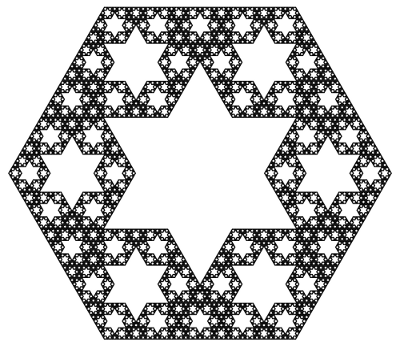Now we are in a position to answer last week’s question: how can we compute the Hausdorff dimension of the hex-fractal? Let d be its dimension. Like last week, our computation will proceed by trying to compute the “d-dimensional volume” of our shape. So, start with a “large” hex-fractal and tri-fractal, each of side-length 1, and let their d-dimensional volumes be h and t respectively.

Break these into “small” hex-fractals and tri-fractals of side-length 1/3, so these have volumes $$h/3^d$$ and $$t/3^d$$ respectively (this is how “d-dimenional stuff” scales). Since $$\begin{gather*}(\text{large hex}) = 6(\text{small hex})+6(\text{small tri}) \quad \text{and}\\ (\text{large tri}) = (\text{small hex})+3(\text{small tri}),\end{gather*}$$ we find that $$h=6h/3^d + 6t/3^d$$ and $$t=h/3^d+3t/3^d$$. Surprisingly, this is enough information to solve for the value of $$3^d$$. We find $$3^d = \frac{1}{2}(9+\sqrt{33})$$, so $$d=\log_3\left(\frac{9+\sqrt{33}}{2}\right) = 1.8184\ldots,$$ as claimed last week.

As a final thought, why did we choose to slice the Menger sponge on this plane? Why not any of the (infinitely many) others? Even if we only look at planes parallel to our chosen plane, a mesmerizing pattern emerges:

It takes a bit more work to turn the above computation of the hex-fractal’s dimension into a full proof, but there are a few ways to do it. Possible methods include mass distributions or similarity graphs.

This diagonal slice through the Menger sponge has been proposed as an exhibit at the Museum of Math. Sebastien Perez Duarte seems to have been the first to slice a Menger sponge in this way (see his rendering), and his animated cross section inspired my animation above.

### Notes

1. We’re assuming that the hex-fractal and tri-fractal have the same Hausdorff dimension. This is true, and it follows from the fact that a scaled version of each lives inside the other. []
2. There are actually two solutions, but the fact that h and t are both positive rules one out. []
3. Proposition 4.9 in: Kenneth Falconer. Fractal Geometry: Mathematical Foundations and Applications. John Wiley & Sons: New York, 1990. []
4. Section 6.6 in: Gerald Edgar. Measure, Topology, and Fractal Geometry (Second Edition). Springer: 2008. []

# A Slice of Interdimensional Sponge Cake

The Menger sponge is a delightfully pathological shape to soak up. Start with a cube of side-length 1, and “hollow it out” by slicing it into 27 sub-cubes (like a Rubik’s Cube) and removing the 7 central sub-cubes as illustrated below. Call this the level 1 sponge. To make the 2nd level, perform the same “hollowing out” operation on each of the 20 cubes in level 1. Keep going to make levels 3, 4, and so on. The end result, i.e., level $$\infty$$, is a fractal known as the Menger Sponge. It has a crazy network of tunnels and passageways running through it: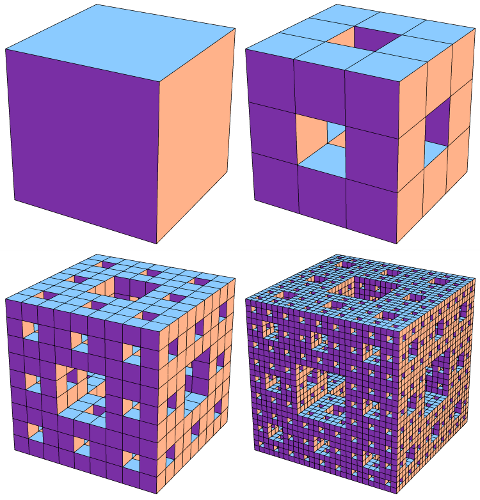Stages in the construction of the Menger sponge: Levels 0, 1, 2, and 3. Click for larger image.

What is its volume? We made the Menger Sponge by cutting out smaller and smaller chunks from the original unit cube, so how much volume did we leave behind? Each “hollowing out” step reduces the volume by a factor of 20/27, so level k has volume $$(20/27)^k$$ and the final Menger Sponge has volume 0. We removed all the volume!

Since the Menger Sponge doesn’t have enough “3D stuff” to be considered a truly 3-dimensional shape, maybe it behaves more like a 2-dimensional surface? This isn’t quite right either. The Menger Sponge has “too much 2D stuff”: its surface area is infinite. In fact, this shape falls squarely (hah!) in the middle: it has dimension 2.7268….

Wait, what does it mean to have non-integer dimension? One interpretation, formalized in the notion of Hausdorff dimension, is to look at how the “stuff” it is made from behaves under scaling. If we take a square (which is made of “2-dimensional stuff”) and scale it by a factor of 1/3, its area changes by a factor of $$(1/3)^2$$. Similarly, scaling a cube (“3D stuff”) by 1/3 scales its volume by $$(1/3)^3$$. In general, scaling “d-dimensional stuff” by a factor of 1/3 scales its “d-dimensional volume” by $$(1/3)^d$$.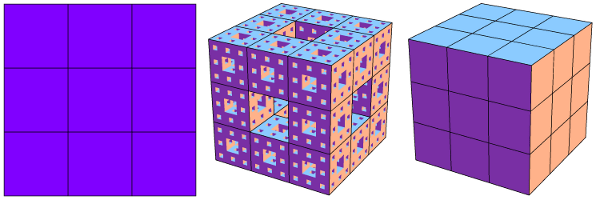How different dimensional "stuff" behaves when scaled by a factor of 1/3. Left: A 2D plane is split into 9=3^2 smaller copies. Right: A 3D cube is split into 27=3^3 copies. Middle: A Menger sponge is split into 20 smaller copies.

As shown in this image, a Menger Sponge can be chopped into 20 smaller Menger Sponges, each scaled by 1/3. So if the Menger Sponge is made of m-dimensional stuff, its m-dimensional volume scales by 1/20 when the shape is scaled by 1/3, so we should have $$(1/3)^m=1/20$$, i.e., $$m=\log_3(20) = 2.7268\ldots$$.

As one more example of the quirkiness that can be squeezed out of the Menger Sponge, what happens when we slice this shape along one of its primary diagonal planes? The Menger Sponge’s crazy network of tunnels creates a stellar array of 6-pointed stars cut out of a regular hexagon: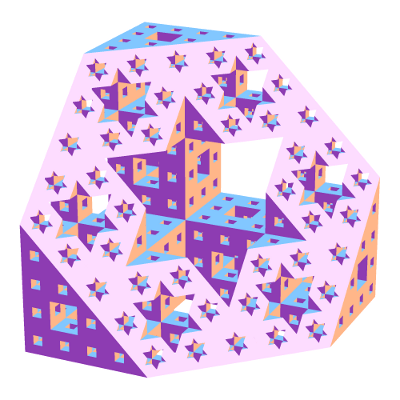Slicing a Menger sponge along a primary diagonal plane produces a dazzling fractal (living in the slicing plane) made from 6-pointed stars.

What is the Hausdorff dimension of this hexagonal fractal? It turns out to be $$\log_3\left(\tfrac{9+\sqrt{33}}{2}\right) = 1.8184\ldots.$$ To find out where this ridiculous number comes from, you’ll have to come back next week!

### Notes

1. This is a technical term, of course. []
2. The surface area of level k can be computed to be $$2\cdot(20/9)^k+4\cdot(8/9)^k$$, and this approaches infinity as k grows. []
3. Specifically, slice it along the perpendicular-bisecting plane of one of the cube’s diagonals []

# Thue-Morse-igami

Here’s a simple origami experiment to try. Start with a long strip of paper with different colors on the two sides—e.g., Orange (O) on one side, Indigo (I) on the other. Now fold the strip in quarters to form a flat “S-shape” as illustrated here (creases are drawn slightly rounded for ease of viewing):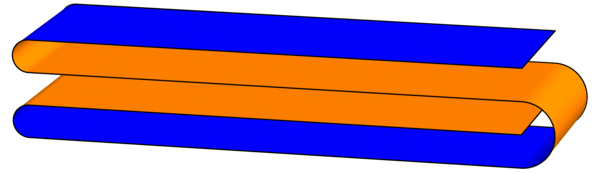As you look through the four layers from top to bottom, write down the colors you encounter, in order: the first layer shows Indigo (I), the second layer has Orange (O), and so on. The full order is IOOI, which looks suspiciously like the start of the Thue-Morse sequence. (This sequence was introduced last week.)

To continue the experiment, treat this figure as a single strip and fold the same flat S-shape again. There will be 16 layers down the middle: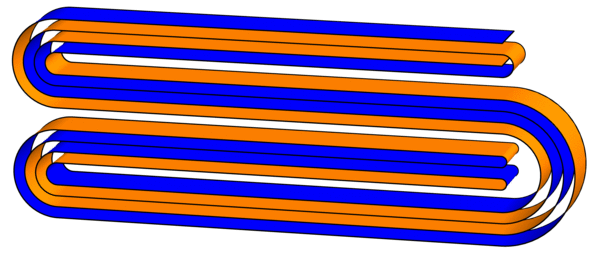Sure enough, the order of colors encountered is IOOI OIIO OIIO IOOI, which is the first 16 digits of the Thue-Morse sequence. This pattern will continue forever (or at least until the paper gets too thick to fold): every time you fold another S-shape, you quadruple the number of digits of the Thue-Morse sequence (can you see why?). Try to fold a third iteration! It looks like this (click for larger image):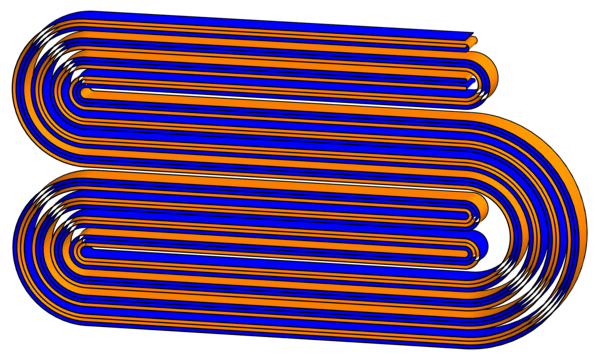But wait, there’s more! Now that we have these creased strips, what happens when we unfold them? Specifically, follow the existing creases and lay out the strips so that each crease makes a 90-degree angle. What shapes do we get? Surprisingly, they exactly fill triangular grids of squares:Can you figure out why this pattern continues?